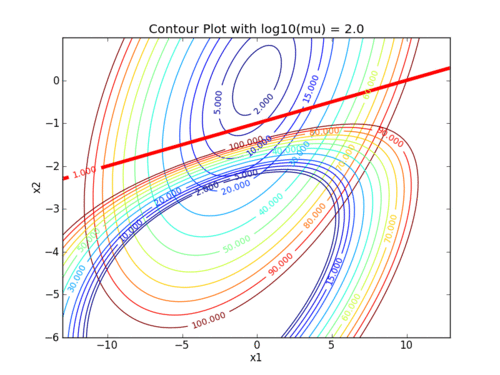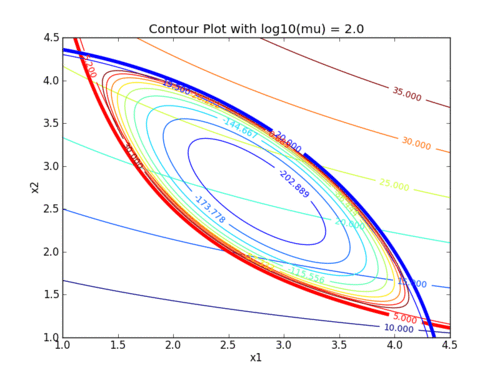## Interior Point Methods

Interior Point Methods are a class of algorithms designed to solve optimization problems. They are used to find the optimal solution of a mathematical optimization problem by moving from one point on the objective function to another point in the interior of the feasible region. Interior Point Methods are often used to solve linear programming problems and can also be used to solve nonlinear programming problems. They typically employ a two-phase approach, with a first phase to find a feasible solution and the second phase to refine the solution to optimality. Interior Point Methods are generally more powerful and efficient than traditional methods, such as the simplex algorithm.

Interior point methods or barrier methods are a certain class of algorithms to solve linear and nonlinear convex optimization problems. Violation of inequality constraints are prevented by augmenting the objective function with a barrier term that causes the optimal unconstrained value to be in the feasible space.

Interior point methods are best suited for very large-scale problems with many degrees of freedom (design variables). Interior point methods are also relatively simple to code into a mathematical program. We will work with interior point methods to investigate the algorithmic details of constrained optimization.

📄 A. Wächter and L. T. Biegler, On the Implementation of an Interior-Point Filter Line-Search Algorithm for Large-Scale Nonlinear Programming, Mathematical Programming 106(1), pp. 25-57, 2006. Download PDF

The difficulty of the last few assignments has been reduced to allow time for work on the Final Project. Please use the additional time this week to develop your project.

#### Interior Point Homework

Two exercises involve setting up and solving nonlinear programming problems with the interior point method.

The following animations demonstrate how the barrier term influences the objective contours. As the value of the barrier term (mu) decreases, the contours of the barrier problem approach the original objective contours.This assignment can be completed in groups of two. Additional guidelines on individual, collaborative, and group assignments are provided under the Expectations link.